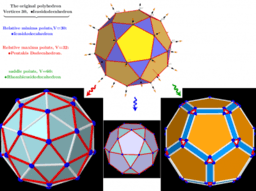# ₆ΛM 3d: Generating uniformly distributed points on a sphere

ΛM -Lagrange Multipliers with One Constraint. Finding Estimators of location on a surface of the sphere as Critical points of the corresponding Lagrangian for a discrete set of points. There is some uniform distribution of points on the sphere. With respect to the points of some other concentric sphere of radius R, we define the distance function f(φ;θ). That is, the sum of all distances from the points of the selected distribution to the (R;φ;θ)- point on this sphere. The critical points: max, min, saddle points of this function form subsets of new "uniform" distributions. And, obviously, a subset of the points of relative minima coincides with the original distribution. Critical points can be found using Lagrange multipliers as finding the Extreme values of the function f(x,y,z) subject to a constraining equation g(x,y,z):=x²+y²+z²-R²=0. There is a system of equations: ∇f(x,y,z)= λ∇g(x,y,z). A local optimum occurs when ∇f(x,y,z) and ∇g(x,y,z) are parallel, and so ∇f is some multiple of ∇g. *From Book: Extended definitions of point location estimates https://www.geogebra.org/m/hhmfbvde From: List of My Public Books on GeoGebra Topics: Constructing polyhedra -https://www.geogebra.org/m/eabstecp